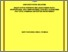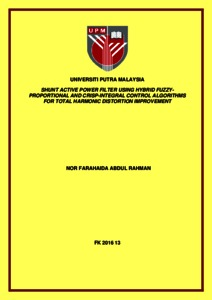# Shunt active power filter using hybrid fuzzy-proportional and crisp-integral control algorithms for total harmonic distortion improvement

## Citation

Abdul Rahman, Nor Farahaida (2016) Shunt active power filter using hybrid fuzzy-proportional and crisp-integral control algorithms for total harmonic distortion improvement. Doctoral thesis, Universiti Putra Malaysia.

## Abstract

Utilization of soft-computing algorithms in the operation of Shunt Active Power Filters (SAPFs) becomes a latest trend. In previous work, an Adaptive Linear Neuron (ADALINE) based extraction algorithm has been developed to generate reference currents. Nevertheless, it has limited function in which it requires a supported conventional synchronization algorithm. On the other hand, Fuzzy-Proportional-Integral (Fuzzy-PI) current and voltage controllers have been introduced in SAPFs’ control system. However, the performance of these controllers depends on simultaneous execution of two input variables and large fuzzy rules. Hence, this complicated structure and operation will affect the capability of SAPFs to work efficiently.Therefore, this work proposes three soft-computing algorithms for generating reference currents, and controlling current and voltage of a three-phase three-leg three-wire SAPF. First, unified ADALINEs based fundamental component extraction algorithm is proposed for dual functionality. It is developed to generate a reference current, and synchronize it with the phase of any operating power system. Hence, the use of conventional synchronization algorithms can be neglected.Second, an adaptive Hybrid Fuzzy-Proportional and Crisp-Integral (HFP+CI) current control algorithm is proposed, by using the indirect control strategy together with an integration of Fuzzy-Proportional (Fuzzy-P) and Crisp-Integral (Crisp-I) current controllers. The Fuzzy-P current controller is used to adaptively tune both current proportional and integral gain values simultaneously, and to perform the proportional control action. Meanwhile, the Crisp-I current controller is developed to perform the integral control action, in which a controllable integral gain value is introduced.Third, a HFP+CI self-charging dc-link voltage control algorithm is constructed, using the self-charging control strategy together with a combination of Fuzzy-P and Crisp-I voltage controllers. In this work, the Fuzzy-P voltage controller is used to adaptively tune only the voltage proportional gain value, and to execute the proportional control action. Subsequently, the Crisp-I voltage controller with a fixed voltage integral gain value is installed.The effectiveness of all the proposed algorithms to perform their respective functions during steady-state and dynamic-state operations is tested, in both simulation and experimental work. Additionally, in simulation work, comparison of the performance of using the proposed HFP+CI, Fuzzy-PI and Crisp-Proportional-Integral (Crisp-PI) current and voltage control algorithms is conducted.According to the shape and the phase of resulted instantaneous supply currents, the proposed extraction algorithm is capable to work as both reference current generator and synchronizer. Other than that, the proposed HFP+CI current control algorithms has resulted better performance with the lowest current ripple, Total Harmonic Distortion (THD) values, and power loss than using Fuzzy-PI and Crisp-PI current control algorithms. Additionally, the proposed HFP+CI voltage control algorithm has resulted fastest response time; it is 94% faster than using the Fuzzy-PI voltage control algorithm and 6% faster than using the Crisp-PI voltage control algorithm.Hence, it can be concluded that the proposed algorithms have successfully performed their functions by effectively enhancing the operation of the SAPF.Preview
Text
FK 2016 13 IR.pdfView Item